SSC  >  SSC CPO Exam Paper 02 July 2017 (Shift-1)

# SSC CPO Exam Paper 02 July 2017 (Shift-1)

Test Description

## 200 Questions MCQ Test | SSC CPO Exam Paper 02 July 2017 (Shift-1)

SSC CPO Exam Paper 02 July 2017 (Shift-1) for SSC 2023 is part of SSC preparation. The SSC CPO Exam Paper 02 July 2017 (Shift-1) questions and answers have been prepared according to the SSC exam syllabus.The SSC CPO Exam Paper 02 July 2017 (Shift-1) MCQs are made for SSC 2023 Exam. Find important definitions, questions, notes, meanings, examples, exercises, MCQs and online tests for SSC CPO Exam Paper 02 July 2017 (Shift-1) below.
Solutions of SSC CPO Exam Paper 02 July 2017 (Shift-1) questions in English are available as part of our course for SSC & SSC CPO Exam Paper 02 July 2017 (Shift-1) solutions in Hindi for SSC course. Download more important topics, notes, lectures and mock test series for SSC Exam by signing up for free. Attempt SSC CPO Exam Paper 02 July 2017 (Shift-1) | 200 questions in 180 minutes | Mock test for SSC preparation | Free important questions MCQ to study for SSC Exam | Download free PDF with solutions
 1 Crore+ students have signed up on EduRev. Have you?
SSC CPO Exam Paper 02 July 2017 (Shift-1) - Question 1

### In the following question, select the related word from the given alternatives. Leaves : Rustle : : ? : ? 1) Rain : Jingle 2) Tyres : Sizzle 3) Clouds : Thunder 4) Snow : Drizzle

SSC CPO Exam Paper 02 July 2017 (Shift-1) - Question 2

### In the following question, select the related word from the given alternatives. Phycology : Algae : : ? : ?

SSC CPO Exam Paper 02 July 2017 (Shift-1) - Question 3

### In the following question, select the related letters from the given alternatives. RVLP : SWMQ : : GLKP : ?

SSC CPO Exam Paper 02 July 2017 (Shift-1) - Question 4

In the following question, select the related letters from the given alternatives.

EFGH : JJJJ : : ADMN : ?

SSC CPO Exam Paper 02 July 2017 (Shift-1) - Question 5

In the following question, select the related number from the given alternatives.

3 : 30 : : 7 : ?

SSC CPO Exam Paper 02 July 2017 (Shift-1) - Question 6

In the following question, select the related number from the given alternatives.

11 : 121 : : 111 : ?

SSC CPO Exam Paper 02 July 2017 (Shift-1) - Question 7

In the following question, select the odd word from the given alternatives.

SSC CPO Exam Paper 02 July 2017 (Shift-1) - Question 8

In the following question, select the odd word pair from the given alternatives.

SSC CPO Exam Paper 02 July 2017 (Shift-1) - Question 9

In the following question, select the odd letters from the given alternatives.

SSC CPO Exam Paper 02 July 2017 (Shift-1) - Question 10

In the following question, select the odd letters from the given alternatives.

SSC CPO Exam Paper 02 July 2017 (Shift-1) - Question 11

In the following question, select the odd number pair from the given alternatives.

SSC CPO Exam Paper 02 July 2017 (Shift-1) - Question 12

In the following question, select the odd number pair from the given alternatives.

SSC CPO Exam Paper 02 July 2017 (Shift-1) - Question 13

Arrange the given words in the sequence in which they occur in the dictionary.

1. First

2. Frown

3. Fist

4. Fast

5. Floor

SSC CPO Exam Paper 02 July 2017 (Shift-1) - Question 14

Arrange the given words in the sequence in which they occur in the dictionary.

1. Hinge

2. Homely

3. Hanger

4. Hasten

5. Howl

SSC CPO Exam Paper 02 July 2017 (Shift-1) - Question 15

A series is given with one term missing. Select the correct alternative from the given ones that will complete the series.

R, M, I, F, D, ?

SSC CPO Exam Paper 02 July 2017 (Shift-1) - Question 16

A series is given with one term missing. Select the correct alternative from the given ones that will complete the series.

K, M, O, Q, ?

SSC CPO Exam Paper 02 July 2017 (Shift-1) - Question 17

In the following question, select the missing number from the given series.

3, 10, 24, 52, 108, ?

SSC CPO Exam Paper 02 July 2017 (Shift-1) - Question 18

In the following question, select the missing number from the given series.

1, 4, 15, 64, ?

SSC CPO Exam Paper 02 July 2017 (Shift-1) - Question 19

Rakesh is 6 ranks ahead of Tanvir in a class of 42 students. If Tanvir's rank is eighteenth from the last, then what is Rakesh's rank from the start?

SSC CPO Exam Paper 02 July 2017 (Shift-1) - Question 20

Aman is 3 years older to Rohit and 3 years younger to Suresh, while Rohit and Danish are twins. How many years is Suresh older to Danish?

SSC CPO Exam Paper 02 July 2017 (Shift-1) - Question 21

Aman, Rohit, Suresh, Danish and Alok are arranged in descending order of weight. Aman is third from the top. Alok is between Danish and Aman while Danish is not at the top. Who is at the top?

SSC CPO Exam Paper 02 July 2017 (Shift-1) - Question 22

In the following question, select the word which cannot be formed using the letters of the given word.

Separated

SSC CPO Exam Paper 02 July 2017 (Shift-1) - Question 23

In the following question, select the word which cannot be formed using the letters of the given word.

Gossiping

SSC CPO Exam Paper 02 July 2017 (Shift-1) - Question 24

In a certain code language, "BLAMED" is written as "DOEGHP" and "DISECT" is written as "VLGWFH". How is "POLARY" written in that code language?

SSC CPO Exam Paper 02 July 2017 (Shift-1) - Question 25

In a certain code language, "HIM-ACE" is written as "21". How is "SIP-TAG" written in that code language?

SSC CPO Exam Paper 02 July 2017 (Shift-1) - Question 26

In the following question, correct the equation by interchanging two numbers.

5 + 3 ÷ 6 × 9 – 2 = 21

SSC CPO Exam Paper 02 July 2017 (Shift-1) - Question 27

If "A" denotes "divided by", "C" denotes "subtracted from", "D" denotes "added to" and "B" denotes "multiplied by", then

15 A 3 D 24 C 12 B 2 = ?

SSC CPO Exam Paper 02 July 2017 (Shift-1) - Question 28

If 62 % 22 ^ 32 = 41 and 72 % 52 ^ 22 = 28, then 52 % 32 ^ 12 = ?

SSC CPO Exam Paper 02 July 2017 (Shift-1) - Question 29

If 6 A 11 B 33 = 18 and 4 B 18 A 9 = 8, then 3 A 5 B 35 = ?

SSC CPO Exam Paper 02 July 2017 (Shift-1) - Question 30

In the following question. select the number which can be placed at the sign of question mark (?) from the given alternatives.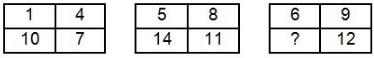SSC CPO Exam Paper 02 July 2017 (Shift-1) - Question 31

In the following question. select the number which can be placed at the sign of question mark (?) from the given alternatives.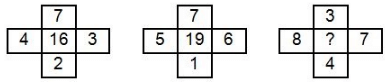SSC CPO Exam Paper 02 July 2017 (Shift-1) - Question 32

How many triangles are there in the given figure?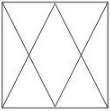SSC CPO Exam Paper 02 July 2017 (Shift-1) - Question 33

How many quadrilaterals are there in the given figure? ​

SSC CPO Exam Paper 02 July 2017 (Shift-1) - Question 34

In each of the following question below are given some statements followed by some conclusions. Taking the given statements to be true even if

they seem to be at variance from commonly known facts, read all the conclusions and then decide which of the given conclusion logically follows the given statements.

Statements:

I. Some pens are glass.

II. All glass are wall.

Conclusions:

I. Some wall are pens.

II. Some wall are glass.

SSC CPO Exam Paper 02 July 2017 (Shift-1) - Question 35

In each of the following question below are given some statements followed by some conclusions. Taking the given statements to be true even if they seem to be at variance from commonly known facts, read all the conclusions and then decide which of the given conclusion logically follows the given statements.

Statements:

I. Some beautiful are intelligent.

II. All intelligent are daughters.

Conclusions:

I. Some intelligent are beautiful.

II. Some daughters are intelligent.

III. Some beautiful are daughters.

SSC CPO Exam Paper 02 July 2017 (Shift-1) - Question 36

From the given options. which answer figure can be formed by folding the figure given in the question?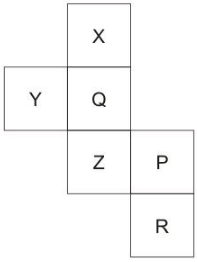SSC CPO Exam Paper 02 July 2017 (Shift-1) - Question 37

From the given options. which answer figure can be formed by folding the figure given in the question?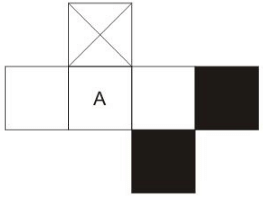SSC CPO Exam Paper 02 July 2017 (Shift-1) - Question 38

In the given figure, which Roman numeral represents the angry kings who are fat?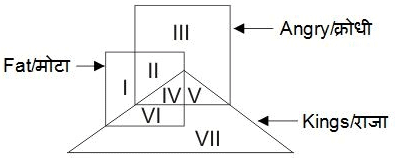SSC CPO Exam Paper 02 July 2017 (Shift-1) - Question 39

In the given figure, how many white apples are there?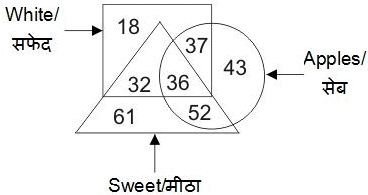SSC CPO Exam Paper 02 July 2017 (Shift-1) - Question 40

In the given figure, which number represents cold drink which is black and sweet?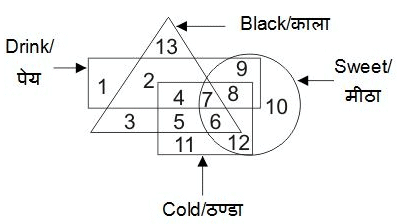SSC CPO Exam Paper 02 July 2017 (Shift-1) - Question 41

Which answer figure will complete the pattern in the question figure?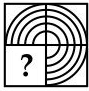SSC CPO Exam Paper 02 July 2017 (Shift-1) - Question 42

Which answer figure will complete the pattern in the question figure?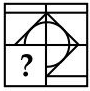SSC CPO Exam Paper 02 July 2017 (Shift-1) - Question 43

From the given answer figures. select the one in which the question figure is hidden/embedded.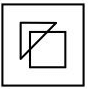SSC CPO Exam Paper 02 July 2017 (Shift-1) - Question 44

From the given answer figures. select the one in which the question figure is hidden/embedded.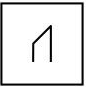SSC CPO Exam Paper 02 July 2017 (Shift-1) - Question 45

A piece of paper is folded and punched as shown below in the question figures. From the given answer figures. indicate how it will appear when opened?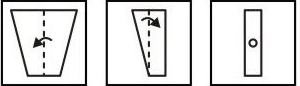SSC CPO Exam Paper 02 July 2017 (Shift-1) - Question 46

A piece of paper is folded and punched as shown below in the question figures. From the given answer figures. indicate how it will appear when opened?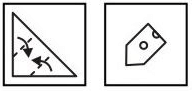SSC CPO Exam Paper 02 July 2017 (Shift-1) - Question 47

If a mirror is placed on the line AB. then which of the answer figures is the right image of the given figure?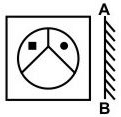SSC CPO Exam Paper 02 July 2017 (Shift-1) - Question 48

If a mirror is placed on the line AB. then which of the answer figures is the right image of the given figure?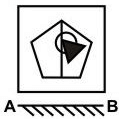SSC CPO Exam Paper 02 July 2017 (Shift-1) - Question 49

A word is represented by only one set of numbers as given in any one of the alternatives. The sets of numbers given in the alternatives are represented by two dasses of alphabets as shown in the given two matrices. The columns and rows of Matrix-I are numbered from 0 to 4 and that of Matrix-II are numbered from 5 to 9 A letter from these matrices can be represented first by its row and next by its column, for example, `G' can be represented by 13, 44, etc., and 'F' can be represented by 67, 86, etc. Similarly, you have to identify the set for the word "MICE".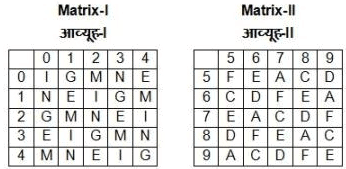SSC CPO Exam Paper 02 July 2017 (Shift-1) - Question 50

A word is represented by only one set of numbers as given in any one of the alternatives. The sets of numbers given in the alternatives are represented by two dasses of alphabets as shown in the given two matrices The colurms and rows of Matrix-I are numbered from 0 to 4 and that of Matrix-fl are numbered from 5 to 9 A letter from these matrices can be represented first by its row and next by its column, for example, 'E' can be represented by 11, 42, etc., and 'N' can be represented by 65, 88, etc. Similarly, you have to identify the set for the word "GRAND".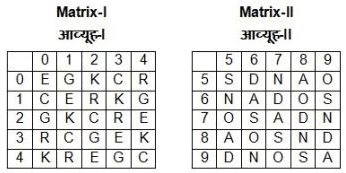SSC CPO Exam Paper 02 July 2017 (Shift-1) - Question 51

Planned economy in India is based on which system?

SSC CPO Exam Paper 02 July 2017 (Shift-1) - Question 52

Planning Commission was constituted in India in which year?

SSC CPO Exam Paper 02 July 2017 (Shift-1) - Question 53

Which one of the following pair is incorrect?

SSC CPO Exam Paper 02 July 2017 (Shift-1) - Question 54

How many Miniratna companies are there in Category – II?

SSC CPO Exam Paper 02 July 2017 (Shift-1) - Question 55

GNP – depreciation allowances = ______

SSC CPO Exam Paper 02 July 2017 (Shift-1) - Question 56

When power is shared among different organs of government, then it known as _____.

SSC CPO Exam Paper 02 July 2017 (Shift-1) - Question 57

Which of the following is CORRECT about coalition form of government?

SSC CPO Exam Paper 02 July 2017 (Shift-1) - Question 58

Which type of government is based on free and fair election where those currently in power have a fair chance of losing?

SSC CPO Exam Paper 02 July 2017 (Shift-1) - Question 59

What is the minimum age required to become a member of Rajya Sabha?

SSC CPO Exam Paper 02 July 2017 (Shift-1) - Question 60

Since Independence, how many time National Emergency has been declared in India?

SSC CPO Exam Paper 02 July 2017 (Shift-1) - Question 61

Who among the following presides over a joint sitting of both the houses summoned by the President of India?

SSC CPO Exam Paper 02 July 2017 (Shift-1) - Question 62

For how much time the Presidents Rule can be extended in India with the approval of Parliament in every six months

SSC CPO Exam Paper 02 July 2017 (Shift-1) - Question 63

What is the minimum number of members required to be present in any one of the two houses of parliament for its functioning?

SSC CPO Exam Paper 02 July 2017 (Shift-1) - Question 64

In Indus Valley Civilization, Kalibangan is famous for which of the following?

SSC CPO Exam Paper 02 July 2017 (Shift-1) - Question 65

Match the following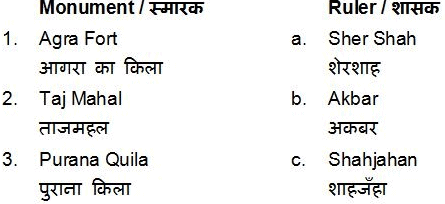SSC CPO Exam Paper 02 July 2017 (Shift-1) - Question 66

Who was the first Governor General of Bengal?

SSC CPO Exam Paper 02 July 2017 (Shift-1) - Question 67

Second battle of Panipat was fought between which two armies?

SSC CPO Exam Paper 02 July 2017 (Shift-1) - Question 68

Who among the following played an important role in Gadar Movement?

SSC CPO Exam Paper 02 July 2017 (Shift-1) - Question 69

Which among the following imaginary line lies at 0° latitude?

SSC CPO Exam Paper 02 July 2017 (Shift-1) - Question 70

Which among the following Indian river flows westwards and falls into Arabian sea?

SSC CPO Exam Paper 02 July 2017 (Shift-1) - Question 71

What are the imaginary lines encircling the earth horizontally called?

SSC CPO Exam Paper 02 July 2017 (Shift-1) - Question 72

From which of the following continent, all three latitudes i.e. equator, tropic of cancer and tropic of Capricorn pass?

SSC CPO Exam Paper 02 July 2017 (Shift-1) - Question 73

Which of the following city is also known as 'zero-mile centre' of India?

SSC CPO Exam Paper 02 July 2017 (Shift-1) - Question 74

Which of the following is an example of Ball and Socket joint in humans?

SSC CPO Exam Paper 02 July 2017 (Shift-1) - Question 75

For which of the following a BT seed has also been developed?

SSC CPO Exam Paper 02 July 2017 (Shift-1) - Question 76

Which of the following contains highest amount of Vitamin C?

SSC CPO Exam Paper 02 July 2017 (Shift-1) - Question 77

Dolly was an example of cloned _____.

SSC CPO Exam Paper 02 July 2017 (Shift-1) - Question 78

Which of the following is a cold blooded animal?

SSC CPO Exam Paper 02 July 2017 (Shift-1) - Question 79

Which of the following glands secret growth hormone?

SSC CPO Exam Paper 02 July 2017 (Shift-1) - Question 80

Jet engine works on the principal of conservation of _____.

SSC CPO Exam Paper 02 July 2017 (Shift-1) - Question 81

What will happen to the level of water in a vessel when a piece of ice floating in it melts?

SSC CPO Exam Paper 02 July 2017 (Shift-1) - Question 82

Which of the following quantity is a measure of inertia?

SSC CPO Exam Paper 02 July 2017 (Shift-1) - Question 83

The instrument used to study the laws of vibrating string is _____.

SSC CPO Exam Paper 02 July 2017 (Shift-1) - Question 84

Which of the following is/are CORRECT?

I. GUI – Graphical User Interface

II. VDU – Visual Display Unit

III. ALU – All Logical Unit

SSC CPO Exam Paper 02 July 2017 (Shift-1) - Question 85

Which among the following is not a word processor?

SSC CPO Exam Paper 02 July 2017 (Shift-1) - Question 86

In which of the following silicon is not used?

SSC CPO Exam Paper 02 July 2017 (Shift-1) - Question 87

Which of the following is used as control rods in Atomic reactor?

SSC CPO Exam Paper 02 July 2017 (Shift-1) - Question 88

Which of the following is used for the preparation of dynamite?

SSC CPO Exam Paper 02 July 2017 (Shift-1) - Question 89

Which among the following is added to commercial nitric acid to make it coloured?

SSC CPO Exam Paper 02 July 2017 (Shift-1) - Question 90

match the following​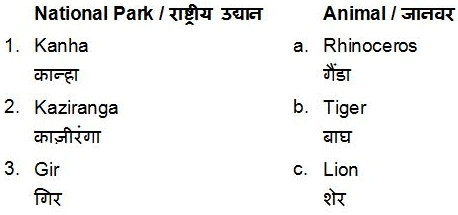SSC CPO Exam Paper 02 July 2017 (Shift-1) - Question 91

Which among the following type of trees are also called as sun loving trees?

SSC CPO Exam Paper 02 July 2017 (Shift-1) - Question 92

Which of the following is an air pollutant?

I. Water vapour

II. Carbon dioxide

III. Hydrogen Gas

SSC CPO Exam Paper 02 July 2017 (Shift-1) - Question 93

Which among the following is an initiative proposed by The Ministry of Civil Aviation to make boarding pass and security check-in digital?

SSC CPO Exam Paper 02 July 2017 (Shift-1) - Question 94

Who amongst the following invented 'chronometer'?

SSC CPO Exam Paper 02 July 2017 (Shift-1) - Question 95

'Calibre' is related to which sport?

SSC CPO Exam Paper 02 July 2017 (Shift-1) - Question 96

All souls day is a _____ festival.

SSC CPO Exam Paper 02 July 2017 (Shift-1) - Question 97

Who is the recipient of Padma Vibhushan 2017 in the field of Art – Music?

SSC CPO Exam Paper 02 July 2017 (Shift-1) - Question 98

Who has authored the book 'The People's Maharaja' based on the life of Amarinder Singh?

SSC CPO Exam Paper 02 July 2017 (Shift-1) - Question 99

Which country has dropped the largest non-nuclear weapon over Afghanistan in April 2017?

SSC CPO Exam Paper 02 July 2017 (Shift-1) - Question 100

Under 'Muktijodha Scholarship Scheme', India will give 35 crore rupees to children of freedom fighters in ______.

SSC CPO Exam Paper 02 July 2017 (Shift-1) - Question 101

If N = (√6 - √5)/(√6 + √5), then what is the value of N + (1/N)?

SSC CPO Exam Paper 02 July 2017 (Shift-1) - Question 102

What is the simplified value of (3 + 1)(32 + 1)(34 + 1)(38 + 1)(316 + 1)?

SSC CPO Exam Paper 02 July 2017 (Shift-1) - Question 103

Which value among √11 + √5, √14 + √2, √8 + √8 is the largest?

SSC CPO Exam Paper 02 July 2017 (Shift-1) - Question 104

What is the value of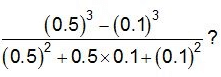SSC CPO Exam Paper 02 July 2017 (Shift-1) - Question 105

How many positive factors of 36 are there?

SSC CPO Exam Paper 02 July 2017 (Shift-1) - Question 106

Two taps A and B can fill a tank in 10 hours and 12 hours respectively. If the two taps are opened at 10 a.m., then at what time (in p.m.) should the tap A be closed to completely fill the tank at exactly 4 p.m.?

SSC CPO Exam Paper 02 July 2017 (Shift-1) - Question 107

Vijay and Sahil together complete a piece of work in 40 days, Sahil and Ranjit can complete the same work in 48 days and Ranjit and Vijay can complete the same work in 60 days. In how many days can all the three complete the same work while working together?

SSC CPO Exam Paper 02 July 2017 (Shift-1) - Question 108

The marked price of an article is 50% more than its cost price. If 20% discount is given, then what will be the profit percentage?

SSC CPO Exam Paper 02 July 2017 (Shift-1) - Question 109

After giving 40% discount on an article there is still a profit of 20%. What will be the profit percentage when no discount is given?

SSC CPO Exam Paper 02 July 2017 (Shift-1) - Question 110

The ratio of two numbers is 4 : 5. If both numbers are increased by 4, the ratio becomes 5 : 6. What is the sum of the two numbers?

SSC CPO Exam Paper 02 July 2017 (Shift-1) - Question 111

If 2A = 3B, then what is the value of (A + B)/A?

SSC CPO Exam Paper 02 July 2017 (Shift-1) - Question 112

The average marks obtained by 180 students in an examination is 50. If the average marks of passed students is 80 and that of the failed students is 40, then what is the number of students who failed the examination?

SSC CPO Exam Paper 02 July 2017 (Shift-1) - Question 113

The average age of four members of a family is 32 years. If the age of a guest is included, then the average age increases by 12.5%. What is the age (in years) of the guest?

SSC CPO Exam Paper 02 July 2017 (Shift-1) - Question 114

If the selling price of 318 articles is equal to the cost price of 212 articles, then what is the loss percentage?

SSC CPO Exam Paper 02 July 2017 (Shift-1) - Question 115

Profit obtained on selling an article for Rs 310 is equal to the loss incurred on selling that article for Rs 230. What will be the loss percentage when selling price is Rs 180?

SSC CPO Exam Paper 02 July 2017 (Shift-1) - Question 116

P is 20% more than Q and 40% less than R. If value of Q is Rs 150, then what is the value of R (in Rs)?

SSC CPO Exam Paper 02 July 2017 (Shift-1) - Question 117

The price of motorcycle depreciates every year by 8%. If the value of the motorcycle after 2 years will be Rs 84640, then what is the present value (in Rs) of the motorcycle?

SSC CPO Exam Paper 02 July 2017 (Shift-1) - Question 118

Diameter of a cycle wheel is 14 cm. A cyclist takes 30 minutes to reach a destination at a speed of 11 km/h. How many revolutions will the wheel make during the journey?

SSC CPO Exam Paper 02 July 2017 (Shift-1) - Question 119

A bus left 60 minutes later than the scheduled time but in order to reach its destination 48 km away in time, it had to increase the speed by 4 km/hr from the usual speed. What is usual speed (in km/hr) of the bus?

SSC CPO Exam Paper 02 July 2017 (Shift-1) - Question 120

If a certain sum becomes 2 times in 7 years at compound interest, then in how many years, it will become 8 times?

SSC CPO Exam Paper 02 July 2017 (Shift-1) - Question 121

A certain sum becomes Rs 1020 in 5 years and Rs 1200 in 8 years at simple interest. What is the value of principal?

SSC CPO Exam Paper 02 July 2017 (Shift-1) - Question 122

The bar graph given below represents the revenue of  a firm for 8 years. All the revenue figures have been shown in terms of Rs crores.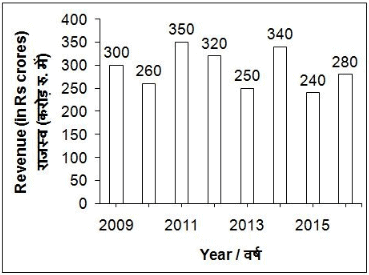Q. What is the total value of revenue of the firm (in crores Rs) in years 2010, 2011 and 2012?

SSC CPO Exam Paper 02 July 2017 (Shift-1) - Question 123

The bar graph given below represents the revenue of  a firm for 8 years. All the revenue figures have been shown in terms of Rs crores.Q. By what percentage has the revenue of the firm decreased in 2010 with respect to the last year?

SSC CPO Exam Paper 02 July 2017 (Shift-1) - Question 124

The bar graph given below represents the revenue of  a firm for 8 years. All the revenue figures have been shown in terms of Rs crores.Q. In how many years, the revenue of the firm is less than the average revenue for these 8 years?

SSC CPO Exam Paper 02 July 2017 (Shift-1) - Question 125

The bar graph given below represents the revenue of  a firm for 8 years. All the revenue figures have been shown in terms of Rs crores.Q. In which year the firm has shown maximum percentage increase in its revenue with respect to the previous year?

SSC CPO Exam Paper 02 July 2017 (Shift-1) - Question 126

If diagonals of a rhombus are 12 cm and 16 cm, then what is the perimeter (in cm) of the rhombus?

SSC CPO Exam Paper 02 July 2017 (Shift-1) - Question 127

Three spherical balls of radius 2 cm, 4 cm and 6 cm are melted to form a new spherical ball. In this process there is a loss of 25% of the material. What is the radius (in cm) of the new ball?

SSC CPO Exam Paper 02 July 2017 (Shift-1) - Question 128

The ratio of curved surface area of two cones is 1 : 8 and the ratio of there slant heights is 1 : 4. What is the ratio of the radius of the two cones?

SSC CPO Exam Paper 02 July 2017 (Shift-1) - Question 129

The perimeter of base of a right circular cone is 44 cm. If the height of the cone is 24 cm, then what is the curved surface area (in cm2) of the cone?

SSC CPO Exam Paper 02 July 2017 (Shift-1) - Question 130

A cuboid which sides 6 cm, 9 cm and 32 cm is melted to form a new cube. What is the ratio between the total surface area of the cuboid and that of the cube?

SSC CPO Exam Paper 02 July 2017 (Shift-1) - Question 131

When a = 61, b = 63 and c = 65, then what is the value of a3 + b3 + c3 - 3abc?

SSC CPO Exam Paper 02 July 2017 (Shift-1) - Question 132

If x = 3 - 2√2, then √x + (1/√x) is equal to ____.

SSC CPO Exam Paper 02 July 2017 (Shift-1) - Question 133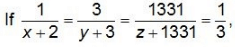then what is value of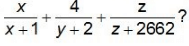SSC CPO Exam Paper 02 July 2017 (Shift-1) - Question 134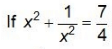for x > 0, then what is the value of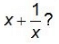SSC CPO Exam Paper 02 July 2017 (Shift-1) - Question 135

If x + y + z = 0, then what is the value of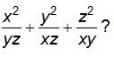SSC CPO Exam Paper 02 July 2017 (Shift-1) - Question 136

What is the ratio of in-radius and circum-radius of an equilateral triangle?

SSC CPO Exam Paper 02 July 2017 (Shift-1) - Question 137

In ΔPQR, ∠P : ∠Q : ∠R = 1 : 3 : 5. What is the value (in degrees) of ∠R - ∠P?

SSC CPO Exam Paper 02 July 2017 (Shift-1) - Question 138

A circular wire of length 168 cm is cut and bent in the form of a rectangle whose sides are in the ratio of 5 : 7. What is the length (in cm) of the diagonal of the rectangle?

SSC CPO Exam Paper 02 July 2017 (Shift-1) - Question 139

In ΔABC, a line parallel to side BC cuts the side AB and AC at points D and E respectively and also point D divide AB in the ratio of 1 : 4. If area of ΔABC is 200 cm2, then what is the area (in cm2) of quadrilateral DECB?

SSC CPO Exam Paper 02 July 2017 (Shift-1) - Question 140

In the given figure. PB is one-third of AB and BQ is one-third of BC. If the area of BPDQ is 20 cm2. then what is the area (in cm2) of ABCD?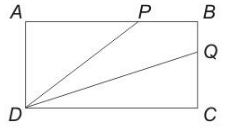SSC CPO Exam Paper 02 July 2017 (Shift-1) - Question 141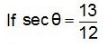and θ is acute, then what is the value of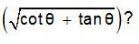SSC CPO Exam Paper 02 July 2017 (Shift-1) - Question 142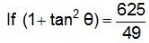and θ is acute, then what is the value of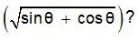SSC CPO Exam Paper 02 July 2017 (Shift-1) - Question 143

If sin θ + sin2 θ = 1, then what is the value of (cos12 θ + 3 cos10 θ + 3 cos8 θ + cos6 θ - 1)?

SSC CPO Exam Paper 02 July 2017 (Shift-1) - Question 144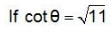and θ is acute, then what is the value of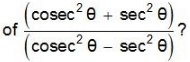SSC CPO Exam Paper 02 July 2017 (Shift-1) - Question 145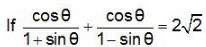and θ is acute, then what is the value (in degrees) of θ?

SSC CPO Exam Paper 02 July 2017 (Shift-1) - Question 146

The given pie chart shows the distribution (in  degrees) of cars sold of different models by a company in 2015-16.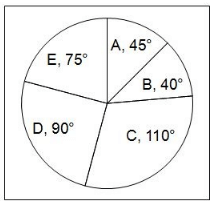Q. If the number of cars sold of model D is 40500, then how many more cars of model E are sold than that of A?

SSC CPO Exam Paper 02 July 2017 (Shift-1) - Question 147

The given pie chart shows the distribution (in  degrees) of cars sold of different models by a company in 2015-16.Q. If the number of cars sold of model D is 40500, what is the ratio between the number of cars sold of model D and E?

SSC CPO Exam Paper 02 July 2017 (Shift-1) - Question 148

The given pie chart shows the distribution (in  degrees) of cars sold of different models by a company in 2015-16.Q. If the number of cars sold of model D is 72900, then what is the total number of cars sold of all the models together by the company?

SSC CPO Exam Paper 02 July 2017 (Shift-1) - Question 149

The given pie chart shows the distribution (in  degrees) of cars sold of different models by a company in 2015-16.Q. If number of cars sold of model C are 22000, then what will be the difference in the number of cars sold of model A and B?

SSC CPO Exam Paper 02 July 2017 (Shift-1) - Question 150

The given pie chart shows the distribution (in  degrees) of cars sold of different models by a company in 2015-16.Q. If 5% of the total number of cars sold of model E is 750, then what is the average number of cars sold of all the models?

SSC CPO Exam Paper 02 July 2017 (Shift-1) - Question 151

In the following question, some part of the sentence may have errors. Find out which part of the sentence has an error and select the appropriate option. If a sentence is free from error, select 'No Error'.

Can you imagine (1)/ that she has thirty (2)/ five pair of shoes. (3)/ No Error (4)

SSC CPO Exam Paper 02 July 2017 (Shift-1) - Question 152

In the following question, some part of the sentence may have errors. Find out which part of the sentence has an error and select the appropriate option. If a sentence is free from error, select 'No Error'.

Mr. Gupta with his wife (1)/ and younger brother (2)/ were present at the station. (3)/ No Error (4)

SSC CPO Exam Paper 02 July 2017 (Shift-1) - Question 153

In the following question, some part of the sentence may have errors. Find out which part of the sentence has an error and select the appropriate option. If a sentence is free from error, select 'No Error'.

Rajni is planning to settle (1)/ in Mumbai as soon as (2)/ she retires in August the next year. (3)/ No Error (4)

SSC CPO Exam Paper 02 July 2017 (Shift-1) - Question 154

In the following question, some part of the sentence may have errors. Find out which part of the sentence has an error and select the appropriate option. If a sentence is free from error, select 'No Error'.

I was born (1)/ on 14th July, 1990 (2)/ in Allahabad. (3)/ No Error (4)

SSC CPO Exam Paper 02 July 2017 (Shift-1) - Question 155

In the following question, some part of the sentence may have errors. Find out which part of the sentence has an error and select the appropriate option. If a sentence is free from error, select 'No Error'.

Not only we saw (1)/ the President but also (2)/ the Chief Minister of Delhi. (3)/ No Error (4)

SSC CPO Exam Paper 02 July 2017 (Shift-1) - Question 156

In the following question, the sentence given with blank to be filled in with an appropriate word. Select the correct alternative out of the four and indicate it by selecting the appropriate option.

Cancer has long been identified as a silent killer because the patient has no _____ of the danger freely invading his system.

SSC CPO Exam Paper 02 July 2017 (Shift-1) - Question 157

In the following question, the sentence given with blank to be filled in with an appropriate word. Select the correct alternative out of the four and indicate it by selecting the appropriate option.

He gave me an additional _____ of Rs 800 a month by permission of the manager.

SSC CPO Exam Paper 02 July 2017 (Shift-1) - Question 158

In the following question, the sentence given with blank to be filled in with an appropriate word. Select the correct alternative out of the four and indicate it by selecting the appropriate option.

Virat Kohli _____ another feather to his cap by his wonderful performance in the T20 World Cup.

SSC CPO Exam Paper 02 July 2017 (Shift-1) - Question 159

In the following question, the sentence given with blank to be filled in with an appropriate word. Select the correct alternative out of the four and indicate it by selecting the appropriate option.

The assistant manager decided to resign when she was _____ for promotion to deputy manager.

SSC CPO Exam Paper 02 July 2017 (Shift-1) - Question 160

In the following question, the sentence given with blank to be filled in with an appropriate word. Select the correct alternative out of the four and indicate it by selecting the appropriate option.

If Neha persists in telling lies to me I shall sue her _____ slander.

SSC CPO Exam Paper 02 July 2017 (Shift-1) - Question 161

In the following question, out of the four alternatives, select the word similar in meaning to the word given.

Epitome

SSC CPO Exam Paper 02 July 2017 (Shift-1) - Question 162

In the following question, out of the four alternatives, select the word similar in meaning to the word given.

Spurious

SSC CPO Exam Paper 02 July 2017 (Shift-1) - Question 163

In the following question, out of the four alternatives, select the word similar in meaning to the word given.

Invincible

SSC CPO Exam Paper 02 July 2017 (Shift-1) - Question 164

In the following question, out of the four alternatives, select the word similar in meaning to the word given.

Trepidation

SSC CPO Exam Paper 02 July 2017 (Shift-1) - Question 165

In the following question, out of the four alternatives, select the word similar in meaning to the word given.

Wastrel

SSC CPO Exam Paper 02 July 2017 (Shift-1) - Question 166

In the following question, out of the four alternatives, select the word opposite in meaning to the word given.

Acknowledge

SSC CPO Exam Paper 02 July 2017 (Shift-1) - Question 167

In the following question, out of the four alternatives, select the word opposite in meaning to the word given.

Abstain

SSC CPO Exam Paper 02 July 2017 (Shift-1) - Question 168

In the following question, out of the four alternatives, select the word opposite in meaning to the word given.

Barbarous

SSC CPO Exam Paper 02 July 2017 (Shift-1) - Question 169

In the following question, out of the four alternatives, select the word opposite in meaning to the word given.

Callow

SSC CPO Exam Paper 02 July 2017 (Shift-1) - Question 170

In the following question, out of the four alternatives, select the word opposite in meaning to the word given.

Eulogistic

SSC CPO Exam Paper 02 July 2017 (Shift-1) - Question 171

In the following question, out of the four alternatives, select the alternative which best expresses the meaning of the idiom/phrase.

At variance with

SSC CPO Exam Paper 02 July 2017 (Shift-1) - Question 172

In the following question, out of the four alternatives, select the alternative which best expresses the meaning of the idiom/phrase.

A snake in the grass

SSC CPO Exam Paper 02 July 2017 (Shift-1) - Question 173

In the following question, out of the four alternatives, select the alternative which best expresses the meaning of the idiom/phrase.

Bolt from the blue

SSC CPO Exam Paper 02 July 2017 (Shift-1) - Question 174

In the following question, out of the four alternatives, select the alternative which best expresses the meaning of the idiom/phrase.

Cry in the wilderness

SSC CPO Exam Paper 02 July 2017 (Shift-1) - Question 175

In the following question, out of the four alternatives, select the alternative which best expresses the meaning of the idiom/phrase.

On the brink of

SSC CPO Exam Paper 02 July 2017 (Shift-1) - Question 176

Improve the bracketed part of the sentence.

SSC CPO Exam Paper 02 July 2017 (Shift-1) - Question 177

Improve the bracketed part of the sentence.

The girl (told her teacher) to explain the passage.

SSC CPO Exam Paper 02 July 2017 (Shift-1) - Question 178

Improve the bracketed part of the sentence.

It will take four hours to walk (across) the caves.

SSC CPO Exam Paper 02 July 2017 (Shift-1) - Question 179

Improve the bracketed part of the sentence.

When I lived in Kanpur I (was going) for movie once a week.

SSC CPO Exam Paper 02 July 2017 (Shift-1) - Question 180

Improve the bracketed part of the sentence.

Abhishek was going to the church (then Neha came on to) meet him.